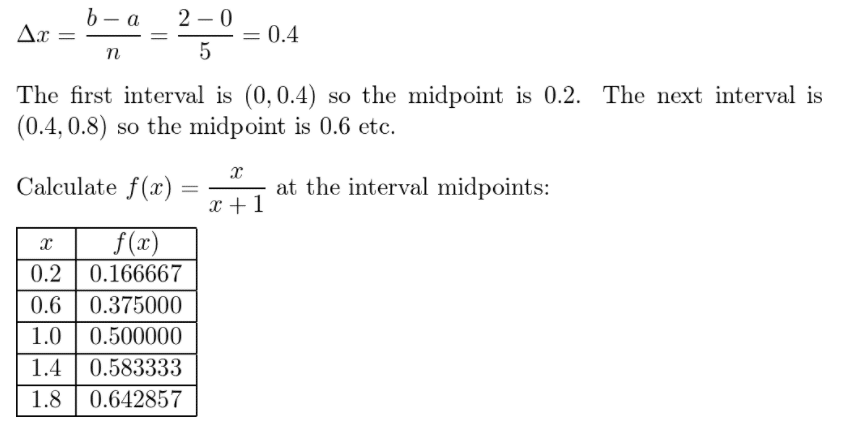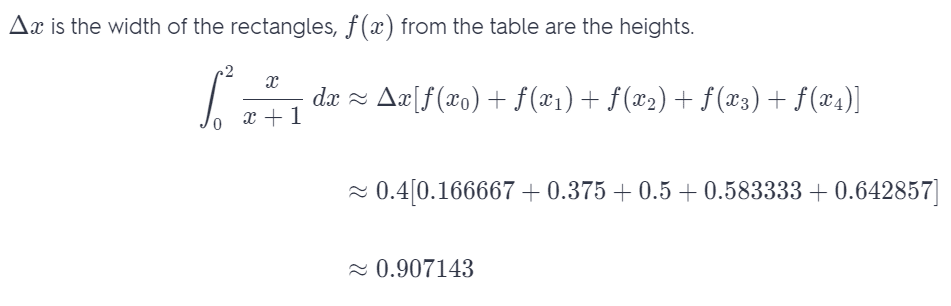# Use The Midpoint Rule With The Given Value Of N To Approximate The Integral

We thoroughly check each answer to a question to provide you with the most correct answers. Found a mistake? Let us know about it through the REPORT button at the bottom of the page.

Use the Midpoint Rule with the given value of n to approximate the integral. Round the answer to four decimal places. ∫2 0 x/x+1 dx, n = 5

In this case, a = 0, b = 2, n = 5.

Find the width of the intervals: